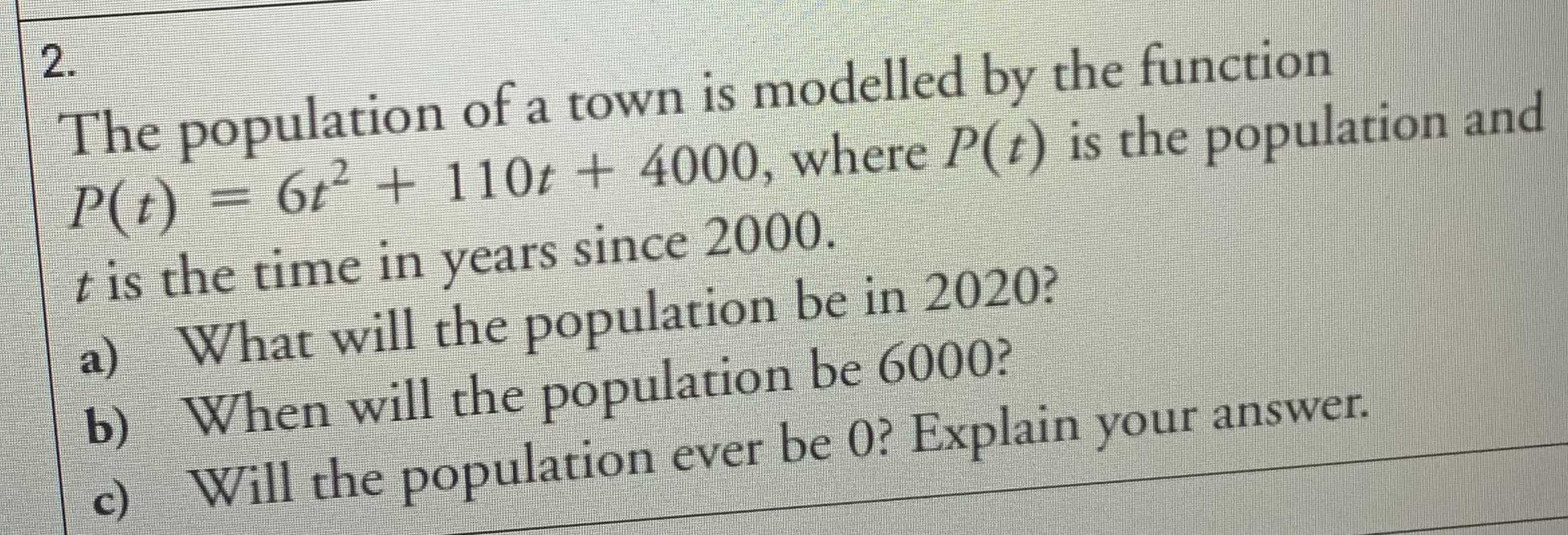### ¿Todavía tienes preguntas de matemáticas?

Pregunte a nuestros tutores expertos
Algebra
Pregunta2. The population of a town is modelled by the function

$$P ( t ) = 6 t ^ { 2 } + 110 t + 4000$$ , where $$P ( t )$$ is the population and $$t$$ is the time in years since $$2000$$ . a) What will the population be in $$2020$$ ? b) When will the population be $$6000$$ ? c) Will the population ever be 0? Explain your answer.

b)t$$\approx 30$$